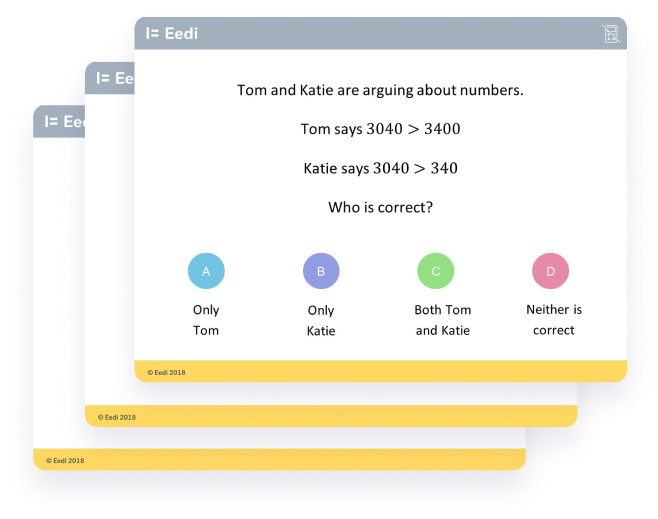# Find links to our full collection of quizzes mapped to some of the most popular maths schemes of work / curricula.

If you have any questions, please email hello@eedi.co.uk.## Diagnostic Questions

Each objective is mapped to a pair of quizzes (Quiz A and Quiz B). Quiz B is a clone of quiz A - it assesses the same mathematical content, but the numbers and the order of answers are changed. You could:
• Use Quiz A in class and set Quiz B for homework
• Set Quiz A for homework, support students in class with the worst answered questions, and then use the questions from Quiz B to see if students have got it
• Set Quiz A as an end of topic assessment, and set Quiz B several weeks later to see what has been retained
• The choice is yours!